# Class 11 Maths NCERT Solutions for Chapter 12 Introduction to Three Dimensional Geometry Exercise 12.3### Three Dimensional Geometry Exercise 12.3 Solutions

1. Find the coordinates of the point which divides the line segment joining the points (–2, 3, 5) and (1, –4, 6) in the ratio (i) 2:3 internally, (ii) 2:3 externally.

Solution

(i) The coordinates of point R that divides the line segment joining points P (x1, y1, z1) and Q (x2, y2, z2) internally in the ratio m: n are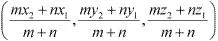Let R (x, yz) be the point that divides the line segment joining points(–2, 3, 5) and (1, –4, 6) internally in the ratio 2:3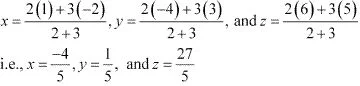Thus, the coordinates of the required point are (-4/5, 1/5, 27/5).

(ii) The coordinates of point R that divides the line segment joining points P (x1, y1, z1) and Q (x2, y2, z2) externally in the ratio m: n are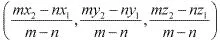Let R(x, y, z) be the point that divides the line segment joining points (-2, 3, 5) and (1, -4, 6) externally in the ratio 2 : 3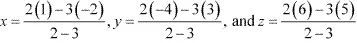i.e., x = -8, y = 17, and z = 3
Thus, the coordinates of the required point are (-8, 17, 3).

2. Given that P (3, 2, –4), Q (5, 4, –6) and R (9, 8, –10) are collinear. Find the ratio in which Q divides PR.

Solution

Let point Q (5, 4, –6) divide the line segment joining points P (3, 2, –4) and R (9, 8, –10) in the ratio k:1.
Therefore, by section formula,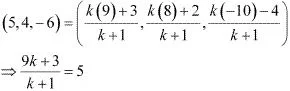⇒ 9k + 3 = 5k + 5
⇒ 4k = 2
⇒ k = 2/4 = 1/2
Thus, point Q divides PR in the ratio 1 : 2.

3. Find the ratio in which the YZ-plane divides the line segment formed by joining the points (–2, 4, 7) and (3, –5, 8).

Solution

Let the YZ planedivide the line segment joining points (–2, 4, 7) and (3, –5, 8) in the ratio k:1.
Hence, by section formula, the coordinates of point of intersection are given by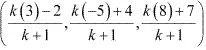On the YZ plane, the x - coordinate of any point is zero.
(3k - 2)/(k + 1) = 0
⇒ 3k -2 = 0
⇒ k = 2/3
Thus, the YZ plane divides the line segment formed by joining the given points in the ratio 2 : 3.

4. Using section formula, show that the points A (2, –3, 4), B (–1, 2, 1) and  C(0, 1/3, 2) are collinear.
Solution

The given points are A (2, –3, 4), B (–1, 2, 1), and C(0, 1/3, 2). .
Let P be a point that divides AB in the ratio k:1.
Hence, by section formula, the coordinates of P are given by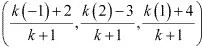Now, we find the value of k at which point P coincides with point C.
By taking (-k + 2)/(k + 1) = 0 , we obtain k = 2.
For k = 2, the coordinates of point P are (0, 1/3, 2).
i.e, C(0, 1/3, 2) is a point that divides AB externally in the ratio 2 : 1 and is the same as point P .
Hence, points A, B, and C are collinear.

5. Find the coordinates of the points which trisect the line segment joining the points P (4, 2, –6) and Q (10, –16, 6).

Solution

Let A and B be the points that trisect the line segment joining points P (4, 2, –6) and Q (10, –16, 6)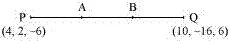Point A divides PQ in the ratio 1 : 2. Therefore, by section formula, the coordinates of point A are given by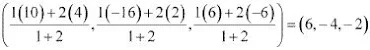Point B divides PQ in the ratio 2 : 1. Therefore, by section formula, the coordinates of point B are given byThus, (6, -4, -2) and (8, -10, 2) are the points that trisect the line segment joining points P(4, 2, -6) and Q(10, -16, 6).